# Propagation of Sound Waves

## Exercise 8(A)

#### Question 1

What causes sound?

Sound is produced when a body vibrates.

#### Question 2

What is sound? How is it produced?

Sound is a form of energy that produces the sensation of hearing in our ears. Sound is produced when a body vibrates.

For example — sound of morning alarm, a dog barking, music from different instruments etc.

#### Question 3

Complete the following sentence —

Sound is produced by a _________ body.

Sound is produced by a vibrating body.

#### Question 4

Describe a simple experiment which demonstrates that the sound produced by a tuning fork is due to vibration of it's arms.

Take a tuning fork which is a rectangular rod of steel bent in U shape, with a metallic stem at the bend. Strike it's one arm on a rubber pad and bring it near a table tennis ball suspended by a thread as shown in the figure below.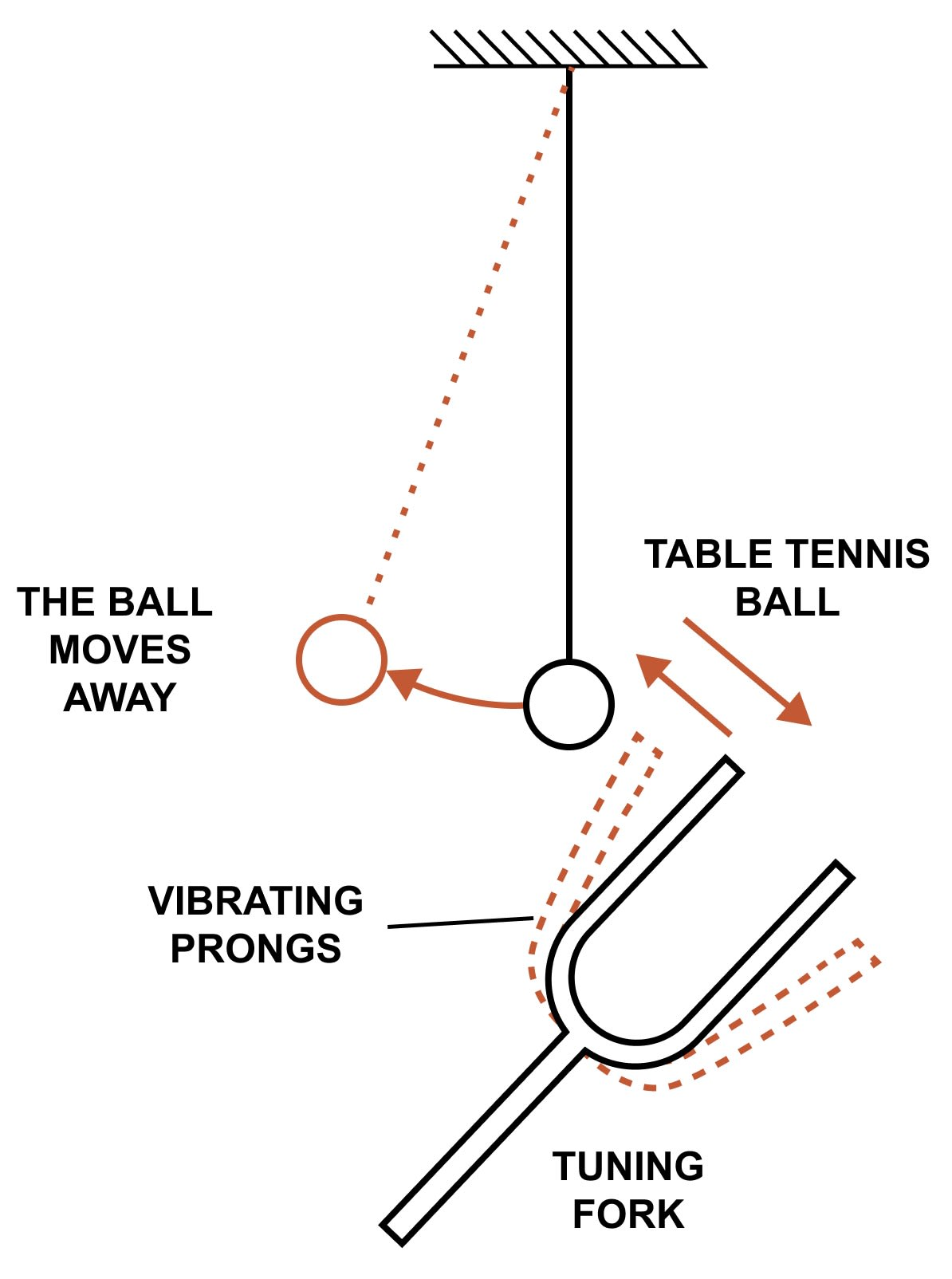It is noticed that as the arm of the vibrating tuning fork is brought close to the ball, the ball jumps to and fro and sound of the vibrating tuning fork is heard. When it's arms stops vibrating, the ball becomes stationary and no sound is heard.

Hence, we can say that the sound produced by a tuning fork is due to vibration of it's arms.

#### Question 5

Describe in brief, with the aid of a labelled diagram, an experiment to demonstrate that a material medium is necessary for the propagation of sound.

A material medium is required for the propagation of sound.

Experiment —

Take an electric bell and an air tight glass bell jar. The electric bell is suspended inside the bell jar. The bell jar is connected to the vacuum pump as shown below.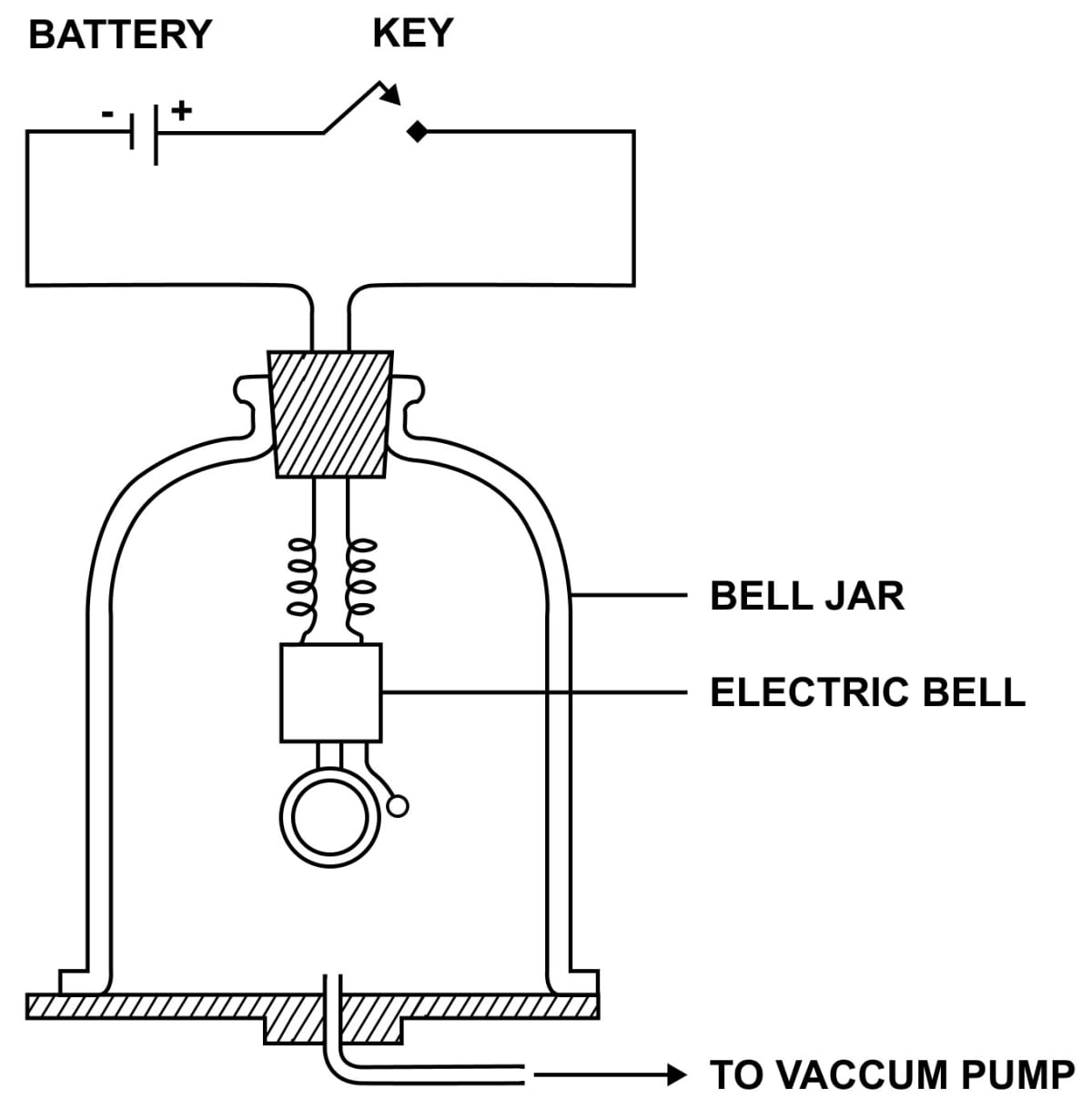As the circuit of electric bulb is completed by pressing the key, the hammer of the electric bell is seen to strike the gong repeatedly and sound of the bell is heard.

Now keeping the key pressed, air is gradually withdrawn from the jar by starting the vacuum pump. It is noticed that the loudness of sound goes on decreasing as the air is taken out from the bell jar and finally no sound is heard when the entire air from the jar has been drawn out.

The hammer of electric bulb is still seen striking the gong repeatedly which means that the gong is still vibrating to produce sound (as hammer strikes the gong), but it is not heard.

Explanation —

When the hammer of the bell hits the gong, sound is produced due to vibration of the gong which travels through the air to the wall of the jar. This causes the wall of jar to vibrate due to which the air outside the jar is also set in vibration.

Thus, sound is heard by us. But when air has been removed from the jar, sound produced due to vibrations of the gong could not travel to the wall of the jar , so wall could not vibrate and no sound is heard.

This clearly demonstrates that sound requires a material medium for it's transmission and it cannot travel through vacuum.

#### Question 6

There is no atmosphere on moon. Can you hear each other on the moon’s surface?

No, we cannot hear each other on the moon's surface because there is no medium for the propagation of sound on moon.

#### Question 7

State three characteristics of the medium required for propagation of sound?

The characteristics of the medium required for propagation of sound in a medium are —

(a) The medium must be elastic so that it's particles may come back to their initial position after displacement on either side, i.e., the particles are capable of vibrating about their mean position.

(b) The medium must have inertia so that it's particles may store mechanical energy.

(c) The medium should be frictionless so that there is no loss of energy in propagation of sound through it.

#### Question 8

Explain with an example, the propagation of sound in a medium.

When a source of sound vibrates, it creates a periodic disturbance in the medium near it. The disturbance then travels in the medium in the form of waves. This can be understood by the following example —

Take a thin metal strip. Keeping it vertical, fix it's lower end. Push it's upper end to one side and then release it. As it vibrates (i.e., moves alternatively to the right and left) sound is heard.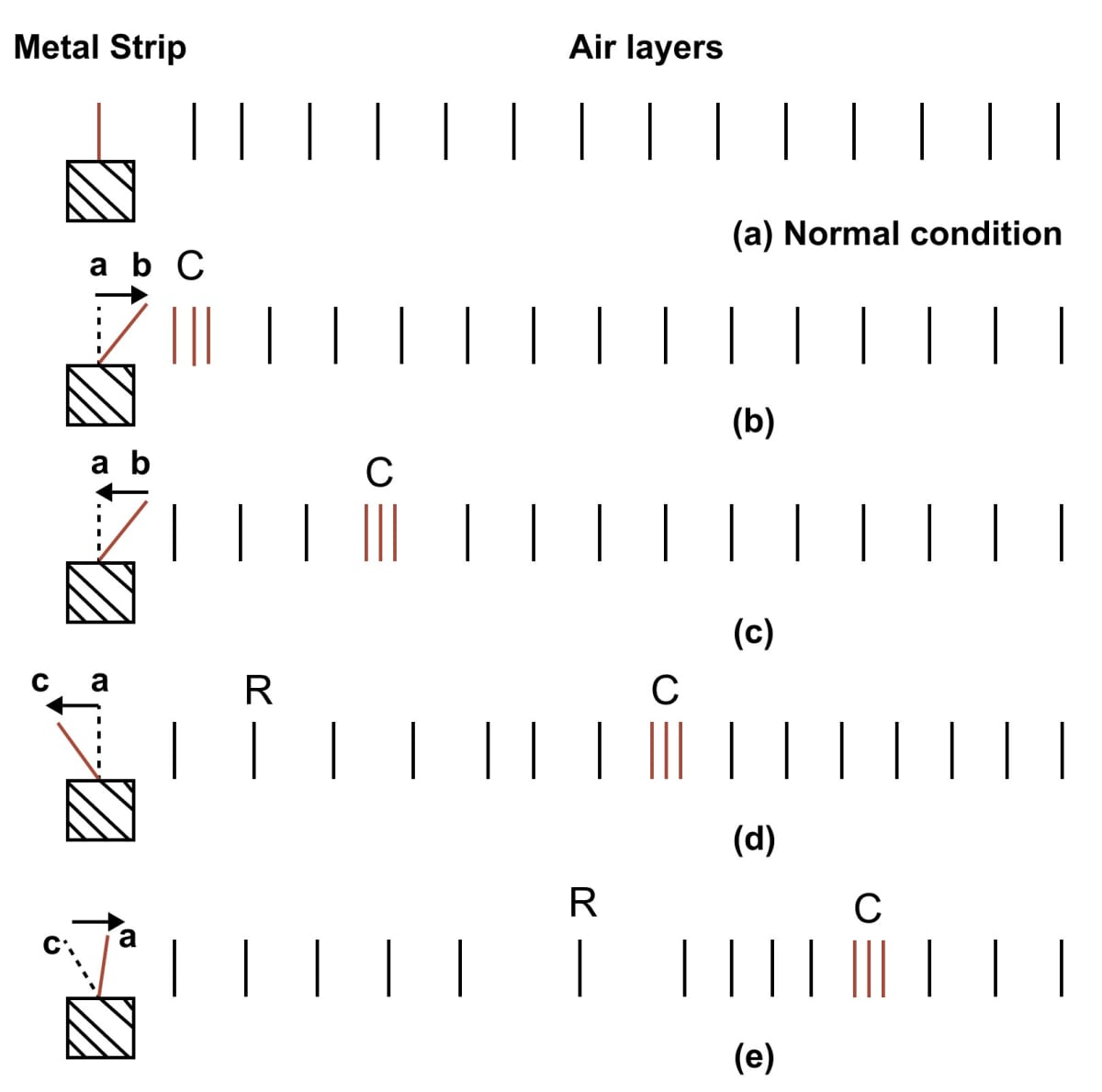When the strip advances to the right from a to b, it pushes the particles of air in layers in front of it. So the particles of air in these layers gets closer to each other i.e., air of these layers gets compressed.

The particles of these layers while moving forward, push and compress the layers next to them, which then compress the next layers and so on. Thus, the disturbance moves forward in form of compression. The particles of the medium get displaced, but they do not move along with the compression.

As the metal strip starts returning from b to a as shown in figure, after pushing the particles in front, the particles of air near the strip starts returning back to their mean positions due to the elasticity of the medium.

When the strip moves to the left from a to c, it pushes back the layers of air near it towards it's left and thus produces a space of very low pressure on it's right side. The air layers on the right side of the strip expand in this region thus forming the rarefied air layers. This region of low pressure is called the rarefaction R.

When the strip returns from c to it's normal position a, it pushes the rarefaction R forward and the air layers near the strip again pass through their mean positions due to the elasticity of the medium.

In this manner, as the strip moves to the right and left repeatedly, the compressions and rarefactions regions are produced one after the other which carry the disturbance with it with a definite speed depending on the nature of the medium. Gradually due to friction, the strip losses its energy to the medium and the disturbance dies out.

One complete to and fro motion of the strip forms one compression and one rarefaction which together constitute one wave. This is how sound waves propagate through a medium such as air.

#### Question 9

Choose the correct word/words to complete the following sentence —

When sound travels in a medium .......... (the particles of the medium, the source, the disturbance, the medium) travels in form of a wave.

When sound travels in a medium the disturbance travels in form of a wave.

#### Question 10

Name the two kinds of waves in form of which sound travels in a medium.

The two kinds of waves in form of which sound travels in a medium are —

1. Transverse waves
2. Longitudinal waves

#### Question 11

What is a longitudinal wave? In which medium: solid, liquid or gas, can it be produced?

The wave in which the particles of medium vibrate about their mean positions, in the direction of propagation of sound is called a Longitudinal wave.

Longitudinal waves can be produced in solids, liquids as well as gases.

#### Question 12

What is a transverse wave? In which medium: solid, liquid or gas, can it be produced?

The wave in which the particles of medium vibrate about their mean positions, in a direction perpendicular to the direction of propagation of the wave is called a Transverse wave.

Transverse waves can only be produced in solids and on the surface of liquids. They cannot be produced inside liquids and in gases.

#### Question 13

Explain the meaning of terms compression and rarefaction in relation to a longitudinal wave.

Compression — When a vibrating object advances, it pushes the particles of air in layers in front of it. So the particles of air in these layers gets closer to each other i.e., air of these layers gets compressed.

The particles of these layers while moving forward, push and compress the layers next to them, which then compress the next layers and so on. Thus, the disturbance moves forward in form of compression.

Rarefaction — When a vibrating object, moves left, it pushes back the layers of air near it towards it's left and thus produces a space near it towards it's right side and thus produces a layer of very low pressure on right side. The air layers on the right side of the strip expand in this region thus forming the rarefied air layers. This region of low pressure is called the rarefaction R.

#### Question 14

Explain the terms crest and trough in relation to a transverse wave.

A transverse wave is composed of a crest and trough. The position of maximum upward displacement is called a crest, while the position of maximum downward displacement is called a trough. The figure below shows crest and trough for a transverse wave: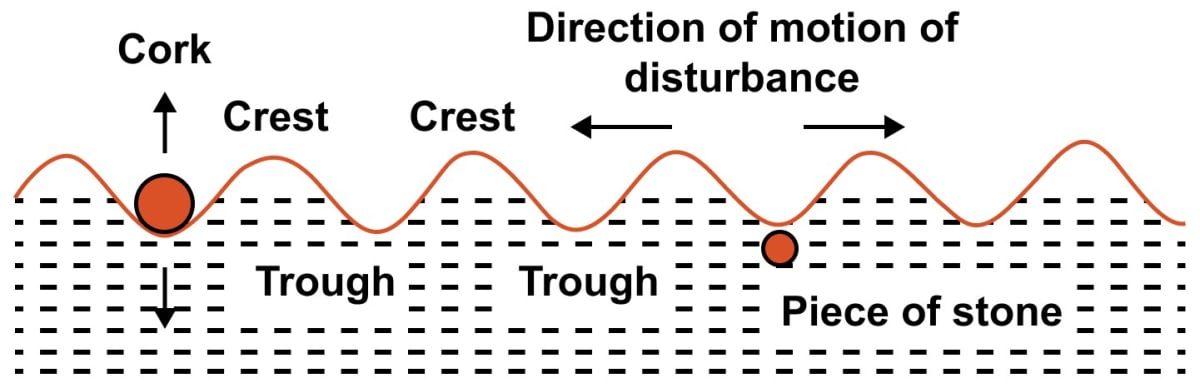#### Question 15

Describe an experiment to show that in wave motion, only energy is transferred, but particles of medium do not leave their positions.

The following experiment shows that in wave motion, only energy is transferred, but particles of medium do not leave their positions.

Experiment

If we drop a piece of stone in the still water of a pond, we hear the sound of stone striking the water surface. A disturbance is produced in water at the point where the stone strikes it. This disturbance spreads in all directions radially outward in form of circular waves on the surface of water as shown in the figure below.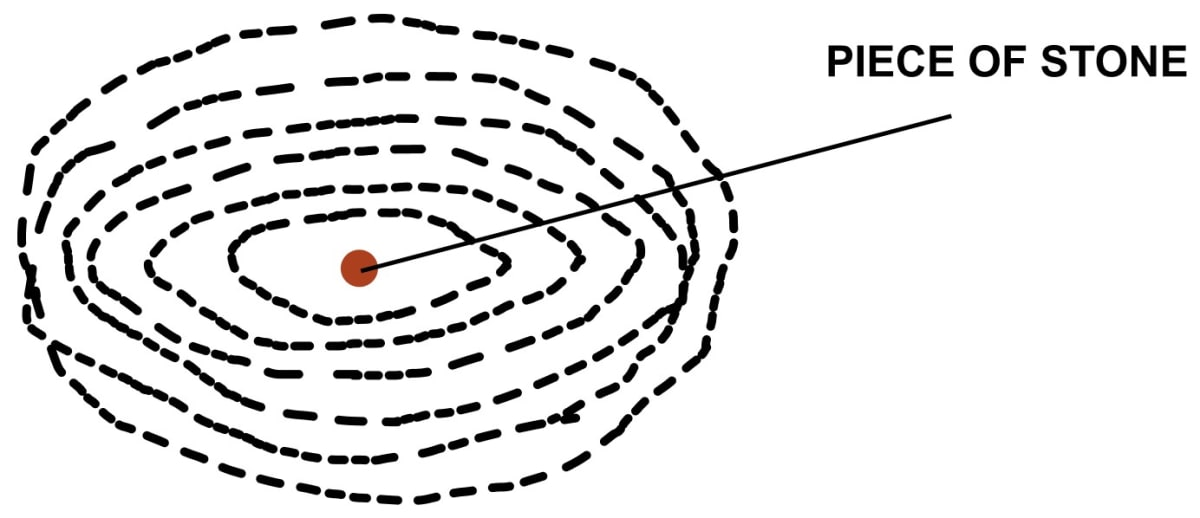Now, if we place a piece of cork on the water surface at some distance away from the point where the stone strikes the water, we notice that the cork does not move ahead, it moves up and down while the wave moves ahead.

The reason is that the cork along with the particles of water (or medium) start vibrating up and down at the point where the stone strikes. These particles then transfer their energy to the other neighbouring particles and they themselves come back to their mean positions. This process continues and thus the disturbance moves ahead on the water surface in form of wave.

Therefore, the above experiment shows that particles do not move but only transfer energy to other particles.

#### Question 16

Define the term amplitude of a wave. Write it's S.I. unit.

When a wave passes through a medium, the maximum displacement of the particle of the medium on either side of it's mean position is called amplitude of wave.

It's S.I. unit is metre (m).

#### Question 17

What do you mean by the term frequency of a wave? State it's S.I. unit.

The number of vibrations made by a particle of a medium in one second is called the frequency of wave. It is the same as the number of waves passing through a point in one second.

It's S.I. unit is second-1 (symbol s-1) or hertz (symbol Hz).

#### Question 18

How is the frequency of a wave related to it's time period?

The frequency (f) and time period (T) are related as

$f = \dfrac{1}{T}$

#### Question 19

Define the term wave velocity. Write it's S.I. unit.

The distance travelled by a wave in one second is called it's wave velocity or wave speed. It is the speed with which energy is transferred from one place to another by wave motion.

It is denoted by the letter V.

It's S.I. unit is metre per second (m s-1).

#### Question 20

Draw displacement-time graph of a wave and show on it the amplitude and time period of wave.

The figure below shows the variation of displacement with time for a particle of the medium at a given position, when a wave propagates through the medium. It is called displacement-time graph.

The amplitude is represented by the letter a and the time period is represented by the letter T.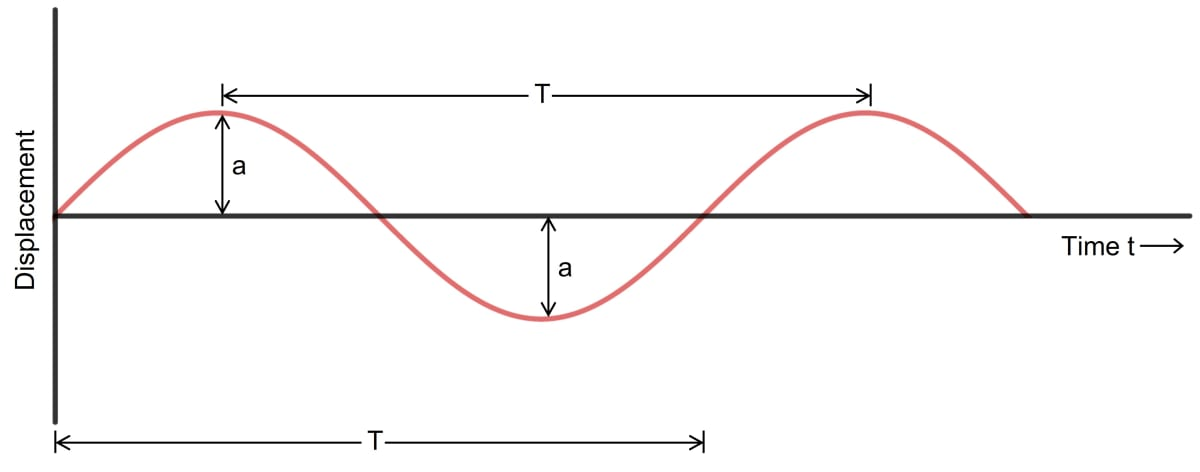#### Question 21

Draw a displacement-distance graph of a wave and mark on it, the amplitude of wave by the letter a and wavelength of wave by the letter λ.

The figure given below shows the displacement-time graph of a transverse wave at an instant. The curve shows the displaced positions at an instant when wave propagates through the medium.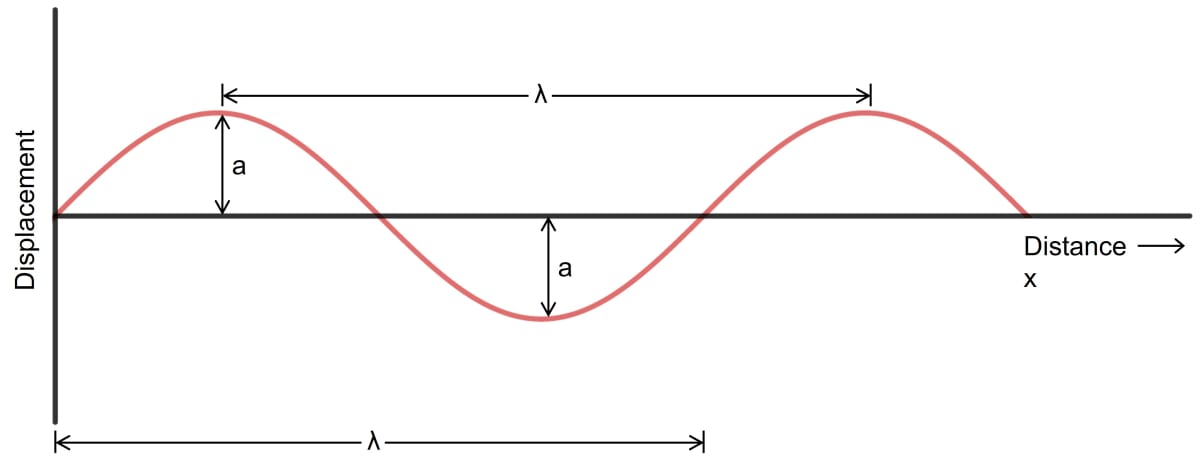#### Question 22

How are the wave velocity V, frequency f and wavelength λ of a wave related? Derive the relationship.

The relation between wave velocity V, frequency f and wavelength λ of a wave is —

V = f λ

Derivation

Let velocity of a wave be V, time period T, frequency f, and wavelength λ.

By definition,

Wavelength λ = Distance travelled by the wave in one time period i.e., in T second
= wave velocity x time period
= V x T
or VT = λ     [Equation 1]

But, T = $\dfrac{1}{f}$

∴ From Equation 1

V x ($\dfrac{1}{f}$) = λ

or V = fλ

Therefore,

Wave velocity (V) = Frequency (f) x Wavelength (λ)

#### Question 23

State two properties of the medium on which the speed of sound in it depends.

Two properties of the medium on which the speed of sound in it depends are —

1. The elasticity (E) of the medium.
2. The density of the medium.

#### Question 24

Arrange the speed of sound in gases Vg, solids Vs and liquids Vl in an ascending order.

Speed of sound in gases Vg, solids Vs and liquids Vl in an ascending order is given below:

Vg < Vl < Vs

#### Question 25

State the speed of (i) light and (ii) sound in air?

(i) The speed of light = 3 x 108 m s-1

(ii) The speed of sound in air = 330 m s-1

#### Question 26

Compare approximately the speed of sound in air, water and steel.

The speed of sound in air is 330 m s-1

The speed of sound in water is 1450 m s-1

The speed of sound in steel is 5100 m s-1

Therefore, the comparison gives —

330 : 1450 : 5100
33 : 145 : 510

So approximately, the speed of sound in air, water and steel are in the ratio 1 : 4 : 15

#### Question 27

(i) Can sound travel in vacuum?
(ii) How does the speed of sound differ in different media?

(i) No, sound cannot travel in vacuum because sound needs a medium to propagate and there are no particles in vacuum to propagate sound.

(ii) The speed of sounds differs in different media. The speed of sound is more in solids, less in liquids and least in gases (since solids are much more elastic than liquids and gases). The speed of sound is nearly 5100 m s-1 in steel, 1450 m s-1 in water and 330 m s-1 in air at 0°C.

#### Question 28

Flash of lightning reaches us earlier than the sound of thunder. Explain the reason.

Flash of lightning reaches us earlier than the sound of thunder because light travels much faster than sound.

Light takes almost negligible time in comparison to sound in reaching us from the place of thunder because speed of light is much more (3 x 108 m s-1) than the speed of sound (= 330 m s-1).

#### Question 29

If you place your ear close to an iron railing which is struck some distance away, you hear the sound twice. Explain why?

As sound travels faster in iron than in air so first the sound through the iron rail is heard and then the sound through the air is heard. That's why we hear the sound twice.

#### Question 30

The sound of an explosion on the surface of a lake is heard by a boat man 100 m away and by a diver 100 m below the point of explosion.

(i) Who would hear the sound first — boat man or diver?
(iii) If sound takes time t to reach the boat man, how much time approximately does it take to reach the diver?

(i) The diver would hear the sound first.

(ii) The sound is heard by the diver first because sound travels faster in water than in air.

(iii) As we know, the speed of sound in water (1450 ms-1 ) is more than the speed of sound in air (330 ms-1) so if 't' is the time taken by sound to reach the boat man, then the time taken by sound to reach the diver is 0.25t as sound travels nearly four times faster in water.

#### Question 31

How do the following factors affect, if at all, the speed of sound in air —

(i) frequency of sound
(ii) temperature of air
(iii) pressure of air
(iv) moisture in air?

(i) Frequency of sound — The speed of sound does not depend on the frequency (or wavelength) of sound wave. Hence, there is no effect on sound when the frequency of sound changes.

(ii) Temperature of air — The speed of sound in a gas increases with an increase in the temperature of the gas.

(iii) Pressure of air — The speed of sound in a gas is independent of pressure. Hence, there is no effect on sound when the pressure of air is changed.

(iv) Moisture in air — The speed of sound in air increases with an increase in the moisture level of air.

#### Question 32

How does the speed of sound change with change in (i) amplitude and (ii) wavelength, of sound wave?

(i) Effect of amplitude of sound wave — The speed of sound does not depend on the amplitude of sound wave. Hence, speed of sound does not change with change in amplitude of sound wave.

(ii) Effect of wavelength of sound wave — The speed of sound does not depend on the wavelength of sound wave. Hence, it does not change with change in wavelength of sound wave.

#### Question 33

In which medium the speed of sound is more — humid air or dry air? Give a reason to your answer.

The speed of sound is more in humid air.

From the relation.

V ∝ $\sqrt\dfrac{\gamma P}{ρ}$, it is clear that V ∝ $\dfrac{1}{\sqrtρ}$ i.e., the speed of sound is inversely proportional to the square root of density of the gas.

As the density of air decreases with an increase in moisture level in air hence speed of sound increases in humid air.

#### Question 34

How does the speed of sound in air vary with temperature?

The speed of sound in a gas increases with the increase in temperature of the gas. The reason is that with the increases in temperature, the density of gas decreases and consequently the speed of sound increases.

In fact, the speed of sound is directly proportional to the square root of temperature of the medium.

The speed of sound in air increases by about 0.61 m s-1 (or 61 cm per second) for each degree celsius rise in temperature (provided that the rise in temperature is not very large).

i.e.,

Vt = V0 + 0.61t

#### Question 35

Describe a simple experiment to determine the speed of sound in air. What approximation is made in the method described by you?

The fact that light travels in air about a million times faster than sound, can be used to determine the speed of sound in air.

Experiment —

Choose two places A and B at high altitudes facing facing each other, at a distance d apart (say, about 1 km), in still iair. The distance d is noted. At each place, there is an observer with a gun and a stop watch. First the observer at place A fires the gun, while the observer at place B starts his stop watch immediately on seeing the flash of fire at A and stops it when he hears the sound of fire. The observer at B by his watch thus finds the time interval t1 taken by the sound to travel from A to B.

Now the observer at place B fires the gun. The observer at A starts his stop watch when he sees the flash of fire at B and stops when he hears the sound of fire. Thus, the observer A by his watch finds the time interval t2 taken by sound to travel from B to A.

The average of the two time intervals is t = $\dfrac{t_1 + t_2 }{2}$. This is the time taken by sound to travel the distance d between the places A and B.

The speed of sound is calculated by using the formula —

V = $\dfrac{\text{Distance}}{\text{Time}}$ = $\dfrac{d}{t}$ m s-1

In the experiment the speed of sound determined is not very accurate because of the personal error of the two observers and the variation in temperature and humidity of air in between the places A and B.

#### Question 36

Complete the following sentences —

(a) Sound cannot travel through _________ ; it requires a _____________

(b) When sound travels in a medium, the particles of medium __________ but the disturbance __________

(c) A longitudinal wave is composed of compression and _____________

(d) A transverse wave is composed of crest and _____________

(e) Wave velocity = ____________ x wavelength

(a) Sound cannot travel through vacuum ; it requires a medium.

(b) When sound travels in a medium, the particles of medium do not move but the disturbance moves ahead.

(c) A longitudinal wave is composed of compression and rarefaction.

(d) A transverse wave is composed of crest and trough.

(e) Wave velocity = frequency x wavelength

## Multiple Choice Type

#### Question 1

The correct statement is:

1. Sound and light both require medium for propagation
2. Sound can travel in vacuum, but light cannot
3. Sound needs medium, but light does not need medium for it's propagation ✓
4. Sound and light both can travel in vacuum

Sound needs medium to propagate hence, sound cannot travel in vacuum, but light does not need medium for it's propagation and so can travel in vacuum.

#### Question 2

The speed of sound in air at 0°C is nearly:

1. 1450 m s-1
2. 450 m s-1
3. 5100 m s-1
4. 330 m s-1

The speed of sound in air at 0°C is 330 m s-1

#### Question 3

Sound in air propagates in form of:

1. Longitudinal wave ✓
2. Transverse wave
3. Both longitudinal and transverse wave
4. Neither longitudinal nor transverse wave

The two kinds of waves in form of which sound travels in a medium are:

(a) Transverse waves — The wave in which the particles of medium vibrate about their mean positions, in a direction perpendicular to the direction of propagation of the wave.

Transverse waves can only be produced in solids and on the surface of liquids. They cannot be produced inside liquids and inside gases.

(b) Longitudinal waves — The wave in which the particles of medium vibrate about their mean positions, in the direction of propagation of sound.

Longitudinal waves can be produced in solids, liquids as well as gases.

Hence, Sound in air propagates in form of longitudinal wave.

#### Question 4

The speed of light in air is:

1. 3 x 108 m s-1
2. 330 m s-1
3. 5100 m s-1
4. 3 x 1010 m s-1

The speed of light in air is 3 x 108 m s-1

## Numericals

#### Question 1

The heart of a man beats 75 times a minute. What is it's (a) frequency and (b) time period?

(a) Given,

Heart beats 75 times a minute

Converting minute into seconds, we get,

1 min = 60 s

As, frequency (f) is the number of times the heart beats in a second

Therefore, we get,

$f = \dfrac{75}{60} \\[0.5em] f = 1.25 \text{ s}^{-1}$

Hence, Frequency = 1.25 s-1

(b) Time period t = $\dfrac{1}{f}$

Substituting the values, we get,

$t = \dfrac{1}{1.25} \\[0.5em] t = 0.8 s$

Hence, t = 0.8 s

#### Question 2

The time period of a simple pendulum is 2 s. Find it's frequency.

Given,

time (t) = 2 s

frequency = ?

As,

Frequency (f) = $\dfrac{1}{t}$

Substituting the values, we get,

$f = \dfrac{1}{2} \\[0.5em] f = 0.5 \text{ Hz}$

Hence, f = 0.5 Hz

#### Question 3

The separation between two consecutive crests in a transverse wave is 100 m. If wave velocity is 20 m s-1, find the frequency of wave.

Given,

wavelength (λ) = 100 m

wave velocity (V)= 20 m s-1

Frequency (f) = ?

As we know,

Wave velocity (V) = Frequency (f) x Wavelength (λ)

Substituting the values, we get,

$20 = f \times 100 \\[0.5em] \Rightarrow f = \dfrac{20}{100} \\[0.5em] f = 0.2 \text{ Hz}$

Hence, f = 0.2 Hz

#### Question 4

A longitudinal wave travels at a speed of 0.3 m s-1 and the frequency of a wave is 20 Hz. Find the separation between the two consecutive compressions.

Given,

Wave velocity (V)= 0.3 m s-1

frequency (f) = 20 Hz

wavelength (λ) = ?

As we know,

Wave velocity (V) = Frequency (f) x Wavelength (λ)

Substituting the values, we get,

$0.3 = 20 \times λ \\[0.5em] \Rightarrow λ = \dfrac{0.3}{20} \\[0.5em] \Rightarrow λ = \dfrac{3}{200} \\[0.5em] \Rightarrow λ = 1.5 \times 10^{-2} \text { m}\\[0.5em]$

Hence, the separation between the two consecutive compressions which is the wave length = 1.5 x 10-2 m ( or 1.5 cm)

#### Question 5

A source of wave produces 40 crests and 40 troughs in 0.4 s. What is the frequency of the wave?

Given,

One crest and one trough make one wave, hence there are 40 waves.

time (t) = 0.4 s

frequency f = ?

Frequency is the number of waves per second,

$f = \dfrac{40}{0.4} \\[0.5em] \Rightarrow f = 100 \text { Hz}$

Hence,

Frequency of the wave = 100 Hz

#### Question 6

An observer A fires a gun and another observer B at a distance 1650 m away from A hears it's sound. If the speed of sound is 330 m s-1, find the time when B will hear the sound after firing by A.

Given,

Distance between A and B = 1650 m

Speed (V)= 330 m s-1

time t = ?

V = $\dfrac{\text{Distance (d)}}{\text {Time (t)}}$

Substituting the values, we get,

$330 = \dfrac{1650}{t} \\[0.5em] \Rightarrow t = \dfrac{1650}{330} \\[0.5em] \Rightarrow t = 5 \text { s} \\[0.5em]$

Therefore, the time when B will hear the sound after firing by A = 5 s.

#### Question 7

The time interval between a lightning flash and the first sound of thunder is 5 s. If the speed of sound in air is 330 m s-1 , find the distance of flash from the observer.

Given,

Time interval (t) = 5 s

Speed (V) = 330 m s-1

distance (d) = ?

V = $\dfrac{\text{Distance (d)}}{\text {Time (t)}}$

Substituting the values, we get,

$330 = \dfrac{d}{5} \\[0.5em] \Rightarrow d = 330 \times 5 \\[0.5em] \Rightarrow d = 1650 \text { m} \\[0.5em]$

Therefore, the distance of flash from the observer = 1650 s.

#### Question 8

A boy fires a gun and another boy at a distance hears the sound of fire 2.5 s after seeing the flash. If speed of sound in air is 340 m s-1 , find the distance between the boys.

Given,

Time (t)= 2.5 s

Speed of sound (V) = 340 m s-1

Distance (d) = ?

V = $\dfrac{\text{Distance (d)}}{\text {Time (t)}}$

Substituting the values, we get,

$340 = \dfrac{d}{2.5} \\[0.5em] \Rightarrow d = 340 \times 2.5 \\[0.5em] \Rightarrow d = 850 \text { m} \\[0.5em]$

Therefore, the distance between the boys = 850 m.

#### Question 9

An observer sitting in line of two tanks, watches the flashes of two tanks firing at each other at the same time, but he hears the sounds of two shots 2s and 3.5s after seeing the flashes. If distance between the two tanks is 510 m, find the speed of sound.

Given,

Time taken to hear the sound of tank 1 = 2 s

Time taken to hear the sound of tank 2 = 3.5 s

Time interval = 3.5 – 2 = 1.5 s

Distance between two tanks = 510 m

Velocity (V) = ?

V = $\dfrac{\text{Distance (d)}}{\text {Time (t)}}$

Substituting the values, we get,

$V = \dfrac{510}{1.5} \\[0.5em] \Rightarrow V = 340 \text { m s}^{-1}\\[0.5em]$

Therefore, the speed of sound = 340 m s-1 .

#### Question 10

How long will sound take to travel in (a) an iron rail and (b) air, both 3.3 km in length? Take speed of sound in air to be 330 m s-1 and in iron to be 5280 m s-1 .

(a) Given,

Length of the iron rail = 3.3 km

Converting km to m, we get,

1 km = 1000 m

Therefore, 3.3 km = 1000 x 3.3 = 3300 m

Speed of the sound in iron (V) = 5280 m s-1

t = ?

V = $\dfrac{\text{Distance (d)}}{\text {Time (t)}}$

Substituting the values, we get,

$5280 = \dfrac{3300}{t} \\[0.5em] \Rightarrow t = \dfrac{3300}{5280} \\[0.5em] \Rightarrow t = 0.625 \text { s} \\[0.5em]$

Therefore, the time taken by sound to travel in iron rail = 0.625 s

(b) Speed of sound in air = 330 m s-1

Substituting the values in the above formula, we get,

$330 = \dfrac{3300}{t} \\[0.5em] \Rightarrow t = \dfrac{3300}{330} \\[0.5em] \Rightarrow t = 10 \text { s} \\[0.5em]$

Therefore, the time taken by sound to travel in air = 10 s

#### Question 11

Assuming the speed of sound in air equal to 340 m s-1 and in water equal to 1360 m s-1 , find the time taken to travel a distance 1700 m by sound in (i) air and (ii) water.

(a) Given,

Speed of sound in air (Va) = 340 m s-1

Speed of sound in water (Vw) = 1360 m s-1

Distance (d) = 1700 m

t = ?

V = $\dfrac{\text{Distance (d)}}{\text {Time (t)}}$

Substituting the values for air, we get,

$340 = \dfrac{1700}{t} \\[0.5em] \Rightarrow t = \dfrac{1700}{340} \\[0.5em] \Rightarrow t = 5 \text { s} \\[0.5em]$

Therefore, the time taken by sound to travel in air = 5 s

(ii) Substituting the values for water, we get,

$1360 = \dfrac{1700}{t} \\[0.5em] \Rightarrow t = \dfrac{1700}{1360} \\[0.5em] \Rightarrow t = 1.25 \text { s} \\[0.5em]$

Therefore, the time taken by sound to travel in air = 1.25 s

## Exercise 8(B)

#### Question 1

What do you mean by the audible range of frequency?

The range of frequency within which the sound can be heard by a human being is called the audible range of frequency.

#### Question 2

What is the audible range of frequency for humans?

The audible range of frequency for humans is 20 Hz to 20 kHz.

#### Question 3

For which range of frequencies are the human ears most sensitive?

The human ears are most sensitive in the range 2000 Hz to 3000 Hz, where it can hear even a very feeble sound.

#### Question 4

Which has a higher frequency — ultrasonic sound or infrasonic sound?

Ultrasonic sound has higher frequency. Sound of frequency above 20,000 Hz is called ultrasonic frequency.

It can travel quite freely in solids and liquids, but in gases it's intensity fails.

#### Question 5

Complete the following sentences —

(a) An average person can hear sounds of frequencies in the range .......... to ..........

(b) Ultrasound is of frequency ..........

(c) Infrasonic sound is of frequency ..........

(d) Bats can produce and hear .......... sound.

(e) Elephants produce .......... sound.

(a) An average person can hear sound of frequencies in the range 20 Hz to 20 kHz

(b) Ultrasound is of frequency above 20 kHz

(c) Infrasonic sound is of frequency below 20 Hz

(d) Bats can produce and hear ultrasonic sound

(e) Elephants produce infrasonic sound

#### Question 6

Name the sounds of the frequencies given below —

(a) 10 Hz

(b) 100 Hz

(c) 1000 Hz

(d) 40 kHz

(a) 10 Hz → Infrasonic sound.

(b) 100 Hz → Audible sound.

(c) 1000 Hz → Audible sound.

(d) 40 Hz → Ultrasonic sound.

#### Question 7

Can you hear the sound produced due to vibrations of a seconds’ pendulum? Give reason.

No, we cannot hear the sound produced due to vibrations of a seconds’ pendulum because the frequency of sound produced due to vibrations of a seconds’ pendulum is 0.5 Hz which is an infrasonic sound and human ears cannot hear infrasonic sound.

#### Question 8

What is ultrasound?

The sound of frequency range of above 20 kHz is called ultrasound.

#### Question 9

State the approximate speed of ultrasound in air.

The approximate speed of ultrasound in air is 330 m s-1 .

#### Question 10

State two properties of ultrasound that make it useful to us.

Properties of ultrasound that make it useful to us are —

1. The energy carried by ultrasound is very high.
2. The ultrasound can travel along a well defined straight path. It does not bend appreciably at the edges of an obstacle because of it's small wavelength (i.e., it has high directivity).

#### Question 11

Explain how do bats locate the obstacles and prey in their way.

Bats avoid obstacles in their path by producing and hearing the ultrasound. They produce ultrasound which returns after striking an obstacle in their way. By hearing the reflected sound, they judge the direction of the obstacle in their way and from the time interval (when they produce ultrasound and then receive them back), they judge the distance of the obstacle.

#### Question 12

State two applications of ultrasound.

The applications of ultrasound are as follows —

1. Ultrasound is used in surgery to remove cataract and in kidney to break the small stones into fine grains.
2. Ultrasound is used for drilling holes or making cuts of desired shapes in materials like glass.

## Multiple Choice Type

#### Question 1

A man can hear the sound of frequency:

1. 1 Hz
2. 1000 Hz ✓
3. 200 kHz
4. 5 MHz

The audible range of frequency for humans is 20 Hz to 20 kHz. Hence, a man can hear the sound of frequency 1000 Hz.

#### Question 2

The properties of ultrasound that make it useful, are:

1. High power and high speed
2. High power and good directivity ✓
3. High frequency and high speed
4. High frequency and bending around the objects

Properties of ultrasound that make it useful to us are:

1. The energy carried by ultrasound is very high.
2. The ultrasound can travel along a well defined straight path. It does not bend appreciably at the edges of an obstacle because of it's small wavelength (i.e., it has high directivity).

#### Question 3

Sonar makes use of:

1. Infrasonic sound
2. Ultrasound ✓
3. Ordinary sound
4. Light